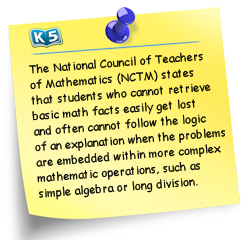# K5 Math Facts

## The goal: instant recall of addition, subtraction, multiplication and division facts

What is 5+ 3? Did the number 8 pop into your head? It should. How about 6 X 7? Instant recall of math facts is when we simply know the answer without having to count in our heads, count fingers or pursue other strategies to reach the answer.

## Why are math facts so important?

Recalling the basic addition, subtraction, multiplication and division facts quickly is critical because it allows a student to study more advanced math topics without being bogged down by simple calculations.

Recall of math facts correlates with success in math at school. Students who don’t have immediate recall of math facts spend much of their time on basic computations instead of learning higher order math skills. Sadly, the average Grade 5 student only knows 32% of his multiplication tables. Both the National Council of Teachers of Mathematics (NCTM) and the National Mathematics Advisory Panel urge that all students develop automatic recall of math facts.

## When to study math facts

Students should begin to focus on developing instant recall of math facts only after they have a firm understanding of the concepts of addition, subtraction, multiplication and division.

Typically, students should strive to have instant recall of their addition and subtraction facts by the end of grade 2 and their multiplication and division facts by the end of grade 3; however, this can vary for each child.  Fluency in math facts will help children gain confidence in math and excel in other areas of math.

## Tools for learning math factsK5's math resources provides materials for both understanding the concepts of addition, subtraction, multiplication and division and for practice of math facts.

Achieving instant recall in math facts is a 3 step process:

1. Gaining a conceptual understanding of the operations (manipulatives, workbooks, worksheets)

2. Solving math fact questions through various strategies until, through repetition, highly competent and confident (worksheets).

3. Memorization of math facts to gain fluency (worksheets, flashcards, online programs or apps)

There are countless apps, games and websites offering math facts practice; however, most of them provide simple random fact  practice.  The best programs analyze chlidrens' responses (both accuracy and speed), evaluate which facts they need additional practice on and adopt their quizzing accordingly, thus making the process quite a bit more efficient.  We recommend XtraMath as a very good and free tool.

## 5 minutes a day to mastery

Developing instant recall in math facts does take time.  Try to practice one addition lesson (5-10 minutes) per day or every second day.  Keeps this up until the student has instant recall of all the math facts up to 10 plus 10.  Then subtraction, multiplication and finally division.